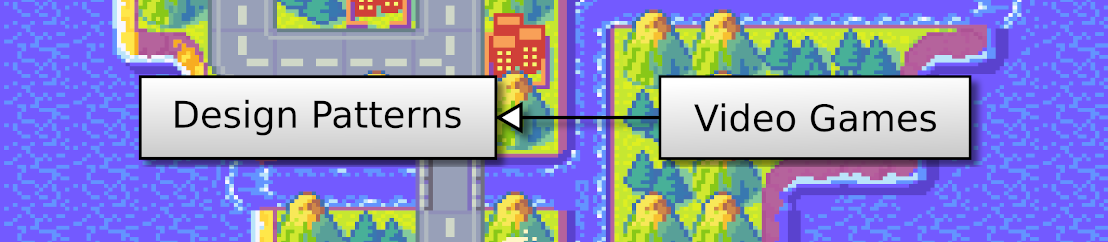## 2D Strategy Game (6): Game logic

We start the design and implementation of the game logic using the Command pattern. Then, we use it to fill areas:

This post is part of the 2D Strategy Game series

We implement game logic with a more advanced Command pattern:

The `Command` interface has three methods, some providing a new feature:

• `priority()`: returns the level of priority. Commands with a low priority value run first. We replace any existing command with the same level. Consequently, we can run only one command with a given priority: this feature can prevent the execution of unnecessary processing. For instance, if all command that changes a cell has the same priority, we only execute the last one.
• `check()`: returns `True` if the command is valid and does something. It is useful when we need to try actions. For example, it can be in the user interface, where we only enable a button if its corresponding command is possible. AI can use this feature to test what it can do.
• `execute()`: the actual processing. We assume that `check()` returned `True` and do the changes with no checks.

Implementation: the `addCommand()` method of the `Logic` class adds the command to the command list. The `executeCommands()` method runs all scheduled commands:

``````def executeCommands(self):
commands = self.__commands.copy()
self.__commands.clear()
priorities = sorted(commands.keys())
for priority in priorities:
command = commands[priority]
if not command.check(self):
continue
command.execute(self)``````

Lines 2-3 copy the list in a variable and clear the attribute. We must proceed this way if the commands schedule commands. Thanks to this copy, we add commands to the attribute, not the list currently processed.

Line 4 sorts the priority levels. The `key()` method returns a list of dictionary keys, and the `sorted()` function returns a sorted list. As a result, `priorities` contains the priority levels from the lowest to the highest.

Line 5 iterates through these levels, and line 6 gets the command corresponding to the current priority. Then, if the `check()` method returns `False`, we end the current iteration (lines 7-8). Finally, we run the command (line 9).

## Commands

We create three command classes to update cells in each layer type (ground, impassable, and objects). These classes inherit a `SetLayerValueCommand` class with shared features:

### Commands priority

The `priority()` method of the `SetLayerValueCommand` class return a priority level that depends on the cell to update:

``````def priority(self) -> int:
return WORLD_PRIORITY + self._coords + self._coords * WORLD_MAX_WIDTH``````

The main idea is to compute a unique integer value for each cell and for the update case. The expression `self._coords + self._coords * WORLD_MAX_WIDTH` is the usual conversion from 2D to 1D coordinates `x + y * width`. We assume that `WORLD_MAX_WIDTH` contains the highest with of the world; this is something we should check when creating or loading a level.

The `WORLD_PRIORITY` value is set to ensure that the priority values don't intersect with others. Right now, these commands are the only ones, so we don't have to worry about that: it will be useful later.

Note that all layer command classes share this `priority()` method: it means that we can only update a cell for a single layer. It is a design choice; if we want to update a cell on several layers simultaneously, we need to create a different set of priority values for each layer.

### Command check

The `check()` method of the `SetGroundValueCommand()` returns `True` if the command leads to an update:

``````def check(self, logic: Logic) -> bool:
value = self._value
if not checkCellValue("ground", value):
return False
coords = self._coords
world = logic.world
if not world.contains(coords):
return False

value = self._value
groundValue = world.ground.getValue(coords)
if value == groundValue:  # Value already set
return False

if value == CellValue.GROUND_SEA:  # Sea case
impassableValue = world.impassable.getValue(coords)
if impassableValue != CellValue.NONE:
return False
objectsValue = world.objects.getValue(coords)
if objectsValue != CellValue.NONE:
return False

return True``````

Lines 2-4 ensure that the value we want to set is correct. For instance, for the ground layer, the possible values are 101 and 102. As before, we don't want to add these values in the code: we collect them in a specific place (the `CellValue` class). Moreover, we also put the logic that checks these values in the same python file with the `checkCellValue()` function:

``````CellValueRanges = {
"ground": (101, 103),
"impassable": (201, 204),
"objects": (301, 311)
}
def checkCellValue(layer: str, value: CellValue):
if layer != "ground" and value == CellValue.NONE:
return True
valueRange = CellValueRanges[layer]
return valueRange <= value < valueRange``````

Note that we also try to write compact code even for implementing this function. For example, we use the `CellValueRanges` dictionary in place of a large `if`...`elif` block to get the value ranges.

The remaining lines of the `check()` method ensure that we don't try to remove ground under an existing element in another layer.

The implementation of the `check()` method in the other command classes is similar.

### Command execution

The `execute()` method of the `SetGroundValueCommand()` sets the value and schedule new commands if we ask for a fill:

``````def execute(self, logic: Logic):
coords = self._coords
value = self._value
ground = logic.world.ground
ground.setValue(coords, value)

if self._fill:
x, y = coords, coords
logic.addCommand(SetGroundValueCommand((x + 1, y), value, True))
logic.addCommand(SetGroundValueCommand((x - 1, y), value, True))
logic.addCommand(SetGroundValueCommand((x, y + 1), value, True))
logic.addCommand(SetGroundValueCommand((x, y - 1), value, True))``````

Lines 8-12 add a command in each direction around the current cell at (x,y). The game logic will execute these commands during the next game epoch. Consequently, if you run the program and click with the middle button, the area around the mouse cursor is slowly filled with a random object. It is because it runs at the pace of the game epoch update, which is 30 times per second (see the end of the main game loop in the `run()` method of `UserInterface`).